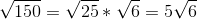## Example Questions

### Example Question #1 : How To Divide Square Roots

(√27 + √12) / √3 is equal to

5/√3

5

(6√3)/√3

18

√3

5

Explanation:

√27 is the same as 3√3, while √12 is the same as 2√3.

3√3 + 2√3 = 5√3

(5√3)/(√3) = 5

### Example Question #2 : How To Divide Square Roots

Simplify: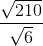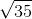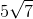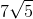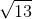Explanation:

When dividing square roots, we divide the numbers inside the radical. Simplify if necessary.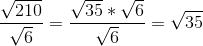### Example Question #3 : How To Divide Square Roots

Simplify: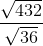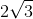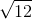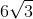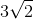Explanation:

When dividing square roots, we divide the numbers inside the radical. Simplify if necessary.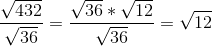Let's simplify this even further by factoring out a.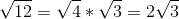### Example Question #4 : How To Divide Square Roots

Simplify: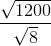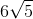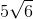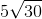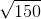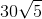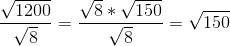Let's simplify this even further by factoring out a.